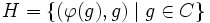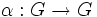# Center not is retraction-invariant

This article gives the statement, and possibly proof, of the fact that for a group, the subgroup obtained by applying a given subgroup-defining function (i.e., center) does not always satisfy a particular subgroup property (i.e., retraction-invariant subgroup)
View subgroup property satisfactions for subgroup-defining functions$|$ View subgroup property dissatisfactions for subgroup-defining functions

## Statement

### Statement with symbols

The center of a group need not be a retraction-invariant subgroup: in other words, there may be a retraction of the whole group under which the center is not mapped to within itself.

## Definitions used

### Center

Further information: Center

The center of a group is defined as the set of all those elements of the group that commute with every element of the group. In symbols, if$G$ is a group, the center of$G$, denoted$Z(G)$, is defined as:$Z(G) = \{ z \in G \mid gz = zg \ \forall \ g \in G \}$.

### Retraction-invariant subgroup

Further information: Retraction,Retraction-invariant subgroup

A retraction of a group is an idempotent endomorphism from the group to itself. In other words, an endomorphism$\alpha$ of a group$G$ is termed a retraction if$\alpha^2 = \alpha$, i.e.,$\alpha(\alpha(g)) = \alpha(g)$ for all$g \in G$.

Retractions arise as follows: Pick a complemented normal subgroup$N$ of$G$ and a permutable complement$H[itex] to [itex]N$ in$G$. In other words,$NH = G$ and$N \cap H$ is trivial. Then, we can define a retraction$\alpha$ of$G$ that sends every element to the unique element of$H$ that lies in the same coset of$N$.

A subgroup$K$ of a group$G$ is termed retraction-invariant if for any retraction$\alpha$ of$G$, we have$\alpha(K) \le K$.

## Proof

### A generic example

Let$A$ be a nontrivial centerless group,$B$ a nontrivial Abelian subgroup of$A$, and$C$ a group isomorphic to$B$ via an isomorphism$\varphi:C \to B$. Define:$G = A \times C$.

Consider the normal subgroup:$N = A \times \{ e \}$

and the complement to it given by:$H = \{ (\varphi(g),g) \mid g \in C \}$.

Let$\alpha:G \to G$ be the retraction with kernel$N$ and image$H$. In other words,$\alpha$ takes any element of$G$ to the unique element of$H$ that is in the same coset of$N$.

Note that$Z(G) = \{ e \} \times C$, because$A$ is centerless and$C$ is Abelian. So,$\alpha(Z(G)) = \alpha( \{ e \} \times C)$. For any$g \in C$, we have that$(\varphi(g),g)$ and$(e,g)$ are in the same coset of$N$. Thus, by definition, we have:$\alpha(e,g) = (\varphi(g),g)$.

Since$\varphi$ is an isomorphism and$C$ is nontrivial, there exists$g \in C$ such that$\varphi(g) \ne e$. But this means that$\alpha(e,g) \notin Z(G)$, indicating that$\alpha(Z(G))$ is not a subgroup of$Z(G)$.